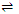Describe physical adsorption and its characteristics.

When the forces of attraction existing between adsorbate and adsorbent are van der Waal's forces, the adsorption is called physical adsorption. This type of adsorption is also known as physisorption or van der Waal's adsorption. Since the forces existing between adsorbent and adsorbate are very weak, therefore, this type of adsorption can be easily reversed by heating or by decreasing the pressure.

Characteristics of Physisorption

Some of the important characteristics of physisorption are as follows:

(i) Deficient of specificity: since the van der Waal forces are universal, a given surface of adsorbent does not show any preference for any specific gas. It can adsorb all the gases but to a different extent.

(ii) Reversible nature: physical adsorption of a gas by the solid is reversible and thus equilibrium is reached rapidly

Solid + Gasgas/solid + Heat

Thus, according to Le-chatelier's principle,

(a) Increase of pressure pushes the equilibrium in forward direction leading to more adsorption of gas and decrease of pressure cause desorption to occur.

(b) Since process is exothermic, therefore, lowering of temperature favours more adsorption and increase of temperature leads to desorption.

(iii) Surface area of adsorbent: the extent of adsorption increase with the increase of surface area of adsorbent. Thus, finely divided metals and rough surfaces are good adsorbents.

(iv) Nature of adsorbate: the amount of gas adsorbed by solid depends on nature of gas. In general, easily liquefiable gases (i.e gases with higher critical temperature) are readily as van der Waal forces are stronger near the critical temperature.

(v) Enthalpy of adsorption: the enthalpy of adsorption is low (20-40 kJ mol-1). This is because of weak nature of van der Waal's forces.

(vii) Activation energy: physical adsorption does not involve any chemical reaction and therefore, it requires very low activation energy.

#### Related Questions in Chemistry

• ##### Q :Molecular Symmetry Types The number of

The number of molecular orbitals and molecular motions of each symmetry type can be deduced. Let us continue to use the C2v point group and the H2O molecule to illustrate how the procedure develop

• ##### Q :Define the term oxidizing agent Briefly

Briefly define the term oxidizing agent?

• ##### Q :Basic concepts Determination of correct

Determination of correct mol. Mass from Roult's law is applicable to :

• ##### Q :Facts on evaporation Illustrate the 3

Illustrate the 3 facts on evaporation?

• ##### Q :Number of electrons in the benzene

Describe the number of electrons in the benzene? Write a short note on it?

• ##### Q :What are Ethers and its types? Ethers

Ethers are the compounds with general formula or CnH2n+

• ##### Q :Why acetic has less conductivity than

Illustrate the reason, why acetic has less conductivity than Hcl?

• ##### Q :Question based on vapour pressure and

Benzene and toluene form nearly ideal solutions. At 20°C, the vapour pressure of benzene is 75 torr and that of toluene is 22 torr. The parial vapour pressure of benzene at 20°C for a solution containing 78g of benzene and 46g of toluene in torr is: (a) 50 (b)

• ##### Q :Short note on the function of

Write down a short note on the function of mitochondria?

• ##### Q :Colligative effect Choose the right

Choose the right answer from following. Which one of the statements written below concerning properties of solutions, explain a colligative effect: (a) Boiling point of pure water decreases by the addition of ethano (b) Vapour pressure of pure water d